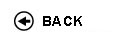Politecnico di Torino
Academic Year 2017/18
02KXWLM
Physics II
1st degree and Bachelor-level of the Bologna process in Computer Engineering - Torino
 Teacher Status SSD Les Ex Lab Tut Years teaching Tresso Elena MariaAC FIS/01 80 20 0 0 7
 SSD CFU Activities Area context FIS/01 6 A - Di base Fisica e chimica
 Subject fundamentals Aim of the course (1st semester, 2nd year) is to provide the students of Informatics Engineering, Telecommunications Engineering, Electronic Engineering and Physical Engineering with the theoretical concepts to be used in the courses of the following semesters. This is therefore a pivotal course for the ensuing career of an ICT engineer. The course is divided in two sections: in the first one, fundamental subjects of basic physics are treated, such as: electromagnetism and the Maxwell's equations, physical and wave/geometrical optics. In the second section, the basic quantum physics concepts needed to describe electronic and optical properties of matter are developed. Expected learning outcomes - Knowledge of magnetostatics. - Ability to apply magnetostatics to simple problems. - Knowledge of basic principles of time-dependent electric and magnetic fields. - Knowledge of Maxwell's equations. - Ability to apply the Maxwell's equations to solve elementary problems of electromagnetism. - Knowledge of wave optics as a consequence of Maxwell's equations. - Knowledge of wave optics laws and of properties of electromagnetic waves. - Ability to apply the laws of wave and geometrical optics e to basic problems and simple optical instruments. - Preliminary Knowledge of laws and principles of quantum mechanics. - Ability to solve elementary problems of quantum mechanics Prerequisites / Assumed knowledge - Basic physics (mechanics, thermodynamics) - Basic mathematics and geometry Contents -Part 1 Electrical currents and magnetostatics (1 cr) Time dependent electric and magnetic fields (1 cr) The Maxwell's equations (0,5 cr) Electromagnetic waves (0,5 cr) Wave optics (0,5 cr) Geometrical optics (0,5 cr) Part 2 Transition from classical to quatum physics. (0,5 cr) The Schroedinger's equation. Measurement of a physical quantity. Indeterminacy principle. (0,5 cr) One-dimensional quantum problems. (1 cr.) Delivery modes Class exercises include simple problem solving activities, with strict connections to theoretical lectures. In some cases scientific calculators (students' personal property) may be required. Texts, readings, handouts and other learning resources Selected chapters from the following textbooks: M. Alonso, E. Finn, Elementi di Fisica per l'Universitŕ Vol. 2, Addison-Wesley 1969 M. Alonso, E. Finn, Fundamental University Physics Vol 3, Addison-Wesley 1968 K.F. Brennan , The Physics of Semiconductors, Cambridge Univ. Press 1999 E.M. Purcell, La Fisica di Berkeley 2 ' Elettricitŕ e magnetismo, Zanichelli 1971 E. H. Wichmann, La Fisica di Berkeley 4 ' Fisica quantistica, Zanichelli 1973 Actual reference texts (selected among those in the list) will be stated by the teacher. Learning material distributed by teacher. Assessment and grading criteria The exam involves a written and an optional oral proof. The written proof includes: a) simple problems (either symbolic or numeric) referring to the main subjects; each problem is articulated in 2-3 points; b) multiple-answer questions on the same subjects of solid state physics. The maximum mark of the problems section is 20/30, that of the questions section is 10/30. The total allotted time is 2 hrs. The written proof is passed with a total score of at least 15/30. The optional oral proof lasts 20-30 mins. and is about all subjects treated in lectures and labs. The final mark is a weighed average of written/oral scores. Notes The course is held by one teacher, responsible for both the first part (4 ECTS) and the second part (2 ECTS). Programma definitivo per l'A.A.2017/18© Politecnico di Torino
Corso Duca degli Abruzzi, 24 - 10129 Torino, ITALY